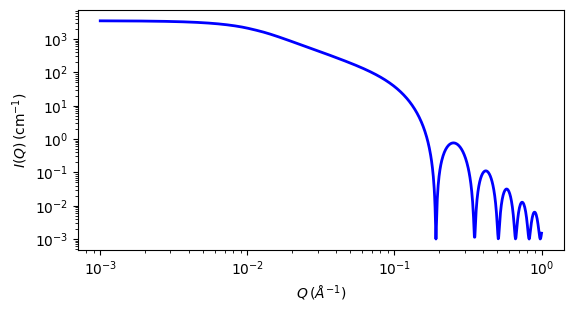# flexible_cylinder

Flexible cylinder where the form factor is normalized by the volume of the cylinder.

Parameter

Description

Units

Default value

scale

Scale factor or Volume fraction

None

1

background

Source background

cm-1

0.001

length

Length of the flexible cylinder

1000

kuhn_length

Kuhn length of the flexible cylinder

100

20

sld

Cylinder scattering length density

10-6-2

1

sld_solvent

Solvent scattering length density

10-6-2

6.3

The returned value is scaled to units of cm-1 sr-1, absolute scale.

This model provides the form factor, $$P(q)$$, for a flexible cylinder where the form factor is normalized by the volume of the cylinder. Inter-cylinder interactions are NOT provided for.

$P(q) = \text{scale} \left<F^2\right>/V + \text{background}$

where the averaging $$\left<\ldots\right>$$ is applied only for the 1D calculation

The 2D scattering intensity is the same as 1D, regardless of the orientation of the q vector which is defined as

$q = \sqrt{q_x^2 + q_y^2}$

Definitions

The chain of contour length, $$L$$, (the total length) can be described as a chain of some number of locally stiff segments of length $$l_p$$, the persistence length (the length along the cylinder over which the flexible cylinder can be considered a rigid rod). The Kuhn length $$(b = 2*l_p)$$ is also used to describe the stiffness of a chain.

In the parameters, the sld and sld_solvent represent the SLD of the cylinder and solvent respectively.

Our model uses the form factor calculations in reference  as implemented in a c-library provided by the NIST Center for Neutron Research (Kline, 2006). This states:

‘Method 3 With Excluded Volume’ is used. The model is a parametrization of simulations of a discrete representation of the worm-like chain model of Kratky and Porod applied in the pseudocontinuous limit. See equations (13,26-27) in the original reference for the details.

Note

There are several typos in the original reference that have been corrected by Chen et al (WRC) . Details of the corrections are in the reference below. Most notably

• Equation (13): the term $$(1 - w(QR))$$ should swap position with $$w(QR)$$

• Equations (23) and (24) are incorrect; WRC has entered these into Mathematica and solved analytically. The results were then converted to code.

• Equation (27) should be $$q0 = max(a3/(Rg^2)^{1/2},3)$$ instead of $$max(a3*b(Rg^2)^{1/2},3)$$

• The scattering function is negative for a range of parameter values and q-values that are experimentally accessible. A correction function has been added to give the proper behavior.

This is a model with complex behaviour depending on the ratio of $$L/b$$ and the reader is strongly encouraged to read reference  before use. In particular, the cylinder form factor used as the limiting case for long narrow rods will not be exactly correct for short and/or wide rods.Fig. 29 1D plot corresponding to the default parameters of the model.

Source

flexible_cylinder.py $$\ \star\$$ flexible_cylinder.c $$\ \star\$$ wrc_cyl.c $$\ \star\$$ sas_J1.c $$\ \star\$$ polevl.c

References

1. J S Pedersen and P Schurtenberger. Scattering functions of semiflexible polymers with and without excluded volume effects. Macromolecules, 29 (1996) 7602-7612

2. W R Chen, P D Butler and L J Magid, Incorporating Intermicellar Interactions in the Fitting of SANS Data from Cationic Wormlike Micelles. Langmuir, 22(15) 2006 6539-6548

Authorship and Verification

• Author: